A Generalized Method for Calculating Phase Matching Conditions inBiaxial Crystals | OMICS International
Journal of Lasers, Optics & Photonics

# A Generalized Method for Calculating Phase Matching Conditions inBiaxial Crystals

Huo G1*, Chen H1 and Zhang M2

1College of Control Engineering, Xijing University, Xi’an, China

2School of Electronics Engineering, Xi’an University of Posts and Telecommunications, Xi’an, China

*Corresponding Author:
Huo G
College of Control Engineering
Xijing University, Xi’an 710123, China
Tel: (8629) 85628198
E-mail: [email protected]

Received Date: November 11, 2016; Accepted Date: December 13, 2016; Published Date: December 30, 2016

Citation: Huo G, Chen H, Zhang M (2016) A Generalized Method for Calculating Phase Matching Conditions in Biaxial Crystals. J Laser Opt Photonics 3: 143. doi: 10.4172/2469-410X.1000143

Copyright: © 2016 Huo G, et al. This is an open-access article distributed under the terms of the Creative Commons Attribution License, which permits unrestricted use, distribution, and reproduction in any medium, provided the original author and source are credited.

Visit for more related articles at Journal of Lasers, Optics & Photonics

#### Abstract

We present a generalized method for calculating phase matching conditions in biaxial crystals, especially in nonlinear monoclinic and triclinic crystals. Exploiting the angle definition introduced by Japanese mathematician Kodaira Kunihiko, we deduce the angular relations in geometry and obtain the expressions of refractive indices depending on angular orientation of wave vector k and optical axis angle. Then we calculate the phase matching parameters with bismuth borate BiB3O6 (BIBO) crystal in spontaneous parametric down conversion (SPDC) process for the type I and type II. On its basis, we discuss the angular gradients of the pump and emission wave refractive index near the exact phase matching direction, and compare the SPDC with double frequency process in geometrical relations of the refractive index ellipsoids. It indicates the anisotropic structures of nonlinear crystals making a capability of filtering effect, and the nonzero linear mismatch described by refractive index angular gradients can be used to estimate the beam width in crystal. This method is convenient to calculate the phase matching parameters in orthorhombic crystals without solving the quadratic Fresnel equations.

#### Keywords

Nonlinear crystals; Phase matching; Angle-dependent refractive index; Spatial and time walk-off

#### Introduction

Since the second-harmonic generation is first found in 1961 , other parameter processes were studied following it, such as sum frequency, difference frequency, optical parametric amplifiers, and optical parametric oscillator. Now, nonlinear frequency conversion has made rapid progress in laser sources owing to its simplicity and wavelength flexibility [2-4]. In 1995, the high efficiency polarization entanglement generation is first demonstrated with BBO crystal . Spontaneous parametric down conversion (SPDC) in nonlinear crystals becomes the mainstay for entanglement production in quantum optics [6,7]. In the process of frequency conversion, phase matching is a key element in improving the conversion efficiency. The imperfect phase matching originates from wave vector direction not coinciding with that of pointing vector inside nonlinear crystal, depressing the conversion efficiency. It results from the detuning of central wavelength and modulation of pump pulse. It is also affected by the energy of pump and emission beams propagating along any phase matching direction with slightly deviation. We need take into account the dielectric dispersion and temperature to calculate accurately the phase matching. Comparing with uniaxial crystal, biaxial crystal, such as Mica, Sapphire, Olivine, Sulfur, has two axes along which the two linearly polarized optical waves have same propagation velocity (or refractive index) [8-11]. Under common conditions in biaxial crystals, two extraordinary linearly polarized beams exist for an incident light, and the refractive index in space distribution are two different ellipsoids . Biaxial nonlinear crystal has excellent optical properties, especially for relatively large effective nonlinear coefficient. Taking the BiB3O6 (BIBO) as an example, it has wide range of optical transparency, high damage threshold, inertness to moisture and the effective nonlinear coefficient attaining 2.5 pm/V [13,14]. These properties make biaxial crystal versatile in phase matching [15-25]. Phase matching in biaxial crystals is first calculated in 1984 , and the improved methods are worked out in the following years [27-30]. All the work was done by solving quadratic Fresnel equations. As the complex optical structure of crystals, a convenient approach for calculating phase matching conditions is worth exploring.

Here, we give out a convenient generalized method for calculating the phase matching parameters of nonlinear frequency conversion in nonlinear crystals, especially for biaxial crystal. In virtue of exploiting the angle definition introduced by Japanese mathematician Kodaira Kunihiko, we obtain the refractive indices contain orientation angles (θ, φ). It can be directly applied in phase matching conditions. Taking SPDC with BIBO crystal as an example, we calculate the effective nonlinear coefficients for type I and type II phase matching. On its basis, we discuss the angular gradients of the pump and emission wave refractive index near the exact phase matching direction, and compare the SPDC with double frequency process in geometrical relations of the refractive index ellipsoids. It indicates the anisotropic structures of nonlinear crystals making a capability of filtering effect, and the nonzero linear mismatch described by refractive index angular gradients can be used to estimate the beam width in crystal. This method is convenient to calculate the phase matching parameters in orthorhombic crystals without solving the quadratic Fresnel equations.

#### The Angular Calculation by Angle Projection in Biaxial Crystals

In biaxial crystals, we can calculate refractive index by wave normal Fresnel equations in principal coordinates system (xyz), while the crystal parameters in fact are measured in crystallographic system (abc). These axes in two different coordinates system are not parallel to each other. In order to find the angle dependence of refractive index, we rotate the principal coordinates system (xyz) φ around z axis, and acquire coordinates x′y′z′; then we rotate the coordinates (x′y′z′) θ around y′axis, obtianing coordinates x"y"z", where θ is polar angle relative to the optical z-axis in plane x′z′, and φ is azimuthal angle measured from x axis in xy plane. The schematic in Figure 1 shows the coordinate transformation of dielectric axis (xyz), crystal principal axis (abc) and lab frame (x"y"z" )The two optical axes (C1, C2) distribute symmetrically about z axis, and the angles between z and C1, C2 are Ω, −Ω respectively. Moreover θ12 are angles between wave vector k and C1, C2, and, θ ′,θ " are angles between z axis and the projections of k in yz and xz planes.

Figure 1: The schematic showing the coordinate transformation of the dielectric axis (xyz), the crystal principal axis (abc), and the lab frame ( x"y"z").

Proved by wave normal Fresnel equations , the refractive indexes of two extraordinary polarized beamsin n1,2 biaxial crystal can be calculated by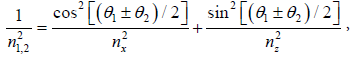(1)

Where nx ny are the indexes in x and z directions. We can get n1 (n2) by taking the upper (lower) sign “+” (“-”) in right side. The angles θ1 and θ2 are key parameters for calculating the refractive index. What’s more, the refractive index exerts an influence on the phase matching conditions in nonlinear frequency conversion, and its derivative decides the birefringence anisotropy in quantum entangled wave packet distribution.

For nonlinear crystals, it is convenient to calculate phase matching parameters in lab frame (x"y"z") during frequency conversion process, while the refractive index is solved easier in crystal’s dielectric axes (xyz). Then, we can seek angular conversion relations between the two coordinates systems in order to utilize the crystal parameters at will. With the method of angle projecting and the angle definition introduced by Japanese mathematician Kodaira Kunihiko , the θ1, θ2 can be expressed below :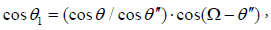(2a)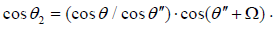(2b)

In Eq (2), θ "=arctan(tanθ. cosφ), and θ ,φ meet the conditions of 0 ≤θ < 90, 0 ≤φ ≤ 90. The optical axis angle can be expressed by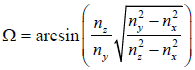where nx, ny and nz are principal refractive indices at a given wavelength . Nevertheless, the Eqs (2) remain correct in the range of and 90 < θ ≤180 and 0° ≤φ ≤ 90° , replacing with θ "=−arctan(tanθ ⋅cosφ ).

From Eqs (1 and 2), the refractive indices are function of Ω ,θ , φ ultimately in monolithic and orthorhombic crystal. The optical axis angle Ω is influenced by wavelength, temperature and so on. The refractive indices here contain two space angles (θ1, θ2). We can take advantage of it to study the characters of phase matching conditions and refractive index angular distribution. The above results are based on monolithic crystal, yet this method can be extended to triclinic crystal through a more angular projecting process, shown in Figure 2. The three crystal axes a, b, c are not vertical to each other in triclinic crystal. We can make b axis projected similarly, decomposed it into two segments which are vertical and parallel to the plane constituted of b and c, separately. According to the cosine relationship cosβ=cosβ ⋅cosβ the angles β1, β2 can be expressed with angles Ω ,θ and φ. We can further obtain the refractive indices in triclinic crystal system through Eq (1 and 2).

#### Phase Matching Conditions of SPDC in Biaxial Crystal

In nonlinear optics, phase matching conditions are crucial to threewave frequency mixing process. It is a complex problem especially in biaxial crystal. At present, the calculation of phase matching in biaxial crystal needs to solve quadratic Fresnel equations. Here we calculate the phase matching conditions with a direct method of angular dependence of refractive index.

In three wave mixing process, it obeys the conservation laws of energy and momentum under phase matching conditions, which meets the relations below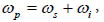(3a)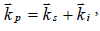(3b)

Where ωp ωs ωi the frequency of pump, signal, and idler beams, respectively. The kp, ks, ki are corresponding wave vectors of the three waves. Generally, there are two beams in biaxial crystal. One is called the ‘‘fast’’ with a smaller refractive index, and the other is named as the ‘‘slow’’ with a bigger one. The momentum conservation in Eq (3) has following forms: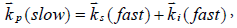(4a)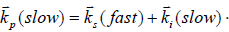(4b)

Eq (4a) is the type I phase matching configuration, and Eq (4b) is the type II.

The wave vectors can be expressed as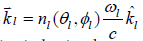(l=p, s, i) for convenience, where n1 is the refractive index in phase matching direction, k1 is the unit vector, and θl, φl are polar and azimuthal angles. The Eq (4) become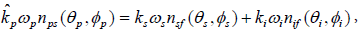(5a)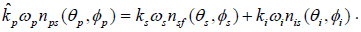(5b)

In Eq (5), the refractive index nl, m(l=p, s, i; m=slow, fast) relies on the phase matching angles (θll) Figure 3 describes the schematic of three wave mixing nonlinear process in biaxial crystal.

For the collinear degenerate SPDC process, the three unit vectors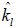are in the same direction, and one incident photon is split into a pair of lower energy correlated photons. The two down-converted photons have equal frequency, satisfying the relation ωisp/2. Then the Eqs (5) are simplified in refractive indices forms .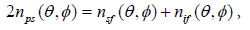(6a)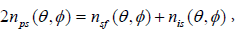(6b)

Where nl,m represent the refractive indices of fundamental and SPDC waves.

The refractive index in Eq (6) at any phase matching direction can be calculated by Eq (1). It is written by: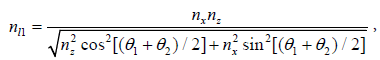(7a)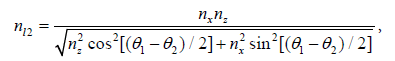(7b)

where l=p, s, i, and θ1, θ2 are deduced in Eq (2) containing the phase matching angles (θ ,φ ). Comparing the two refractive indices in Eqs. (7), the smaller (bigger) one is the “fast” (“slow”) indicated as nlf (nlf).

BIBO is a new type biaxial crystal with good optical properties. Using the parameters from Hellwig’s data at room temperature , the dispersion curves are plotted in Figure 4. From the dispersion relation in Figure 4, we can get the Sellmeier coefficients at arbitrary wavelength.

Combining Eq (6) and Eq (7) together, we can get the relations of phase matching angles (θ ,φ ) in SPDC process in the range of θ = 0° − 90° and θ = 0° − 90°. Figure 5a shows the type I phase matching angles at fundamental wavelength of 400nm, while Figure 5b describes the type II at 800nm. It is fundamental to calculate the phase matching characters, such as the effective nonlinear coefficients, the spatial walk-off angle, acceptance angle, spectral acceptance, and group velocity dispersion.

Figure 5: The relations of phase matching angles in SPDC process, with φ = 0° − 90° , θ = 0° − 90° , (a) the type I at fundamental wavelength of 400 nm, and (b) the type II at 800 nm.

#### The Superiority of Angular-Dependent Refractive Index

From the discussion above, we solve the linear relations of refractive indices in phase matching conditions, avoiding complex quadratic Fresnel equations. It is a convenient method for calculating the phase matching parameters, especially for biaxial crystal. We can see that the spatial walk-off angles are bigger for type II phase matching, depressing the efficiency of SPDC. Thus, the angle acceptance and spectral acceptance are larger than that of type I, which is good for improving the efficiency. These results indicate that the type II phase matching in SPDC process with BIBO crystal is a good choice. Owing to afore mentioned monoclinic symmetry, the distributions of the effective nonlinear coefficient are symmetrical around the axes φ=0, φ=90 and φ=−90 . We can obtain the optimal phase matching conditions easily in other principle planes.

The specified phase matching parameters here are acquired by taking advantage of angular-dependent refractive indices of biaxial crystals in Eq (7). In the near axis and wide crystal approximation, the function n(θ ,φ) can be approximated by the zero and first orders of its expansion. The linear detuning is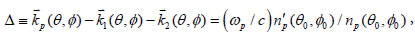(8)

Where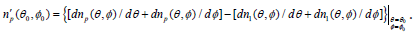(9)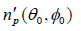is the gradient of refractive index at (θ00) and the linear detuning Δ does not equal zero.

The angular gradient of pump wave refractive index n′p can be used to explain three wave interactions both in and out of principal planes. It is easy to ascertain the directions of pump and emission waves by two refractive index ellipsoids. More importantly, the linear detuning offered a method for describing the beam width in biaxial crystals, affording a quantification parameter to estimate the space distribution of pump and emission waves in quantum optics.

#### Conclusion

In conclusion, we have presented an effective method for calculating phase matching with biaxial crystals in three-wave frequency mixing. The refractive indices depending on angular orientation (θ,φ) can be directly applied in common phase matching conditions without solving quadratic Fresnel equations. Taking collinear SPDC in BIBO for an example, we calculated the phase matching angles for the type I and type II based on angle-dependent refractive index. It is fundamental for calculating all the other phase matching parameters, such as the effective nonlinear coefficients, the spatial and time walkoff. Moreover, the angular gradient of refractive index near the phase matching direction can be used to decide the optimum phase matching conditions and the space distribution of pump and emission waves. This method is convenient to calculate the phase matching parameters in orthorhombic crystals, and it can be used to estimate the beam width in crystal.

#### Acknowledgments

The authors thank the Xijing University Research Foundation for Talented Scholars (No. XJ15B02), and the Scientific Research Program Funded by Shaanxi Provincial Education Department (No. 16JK2247).

#### References

Select your language of interest to view the total content in your interested language

### Article Usage

• Total views: 2308
• [From(publication date):
December-2016 - Dec 11, 2019]
• Breakdown by view type
• HTML page views : 2136Can't read the image? click here to refresh